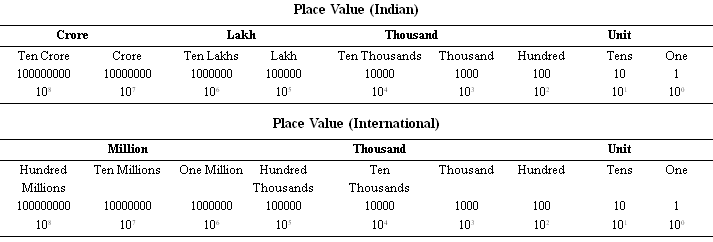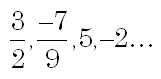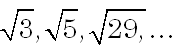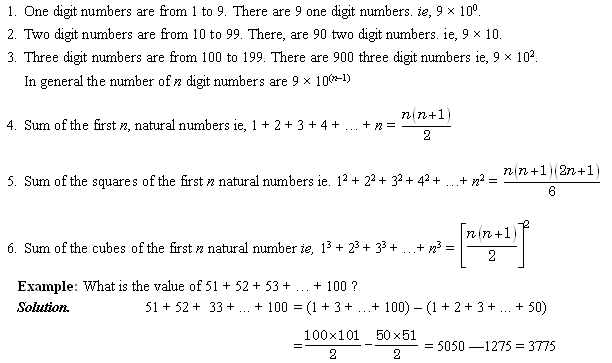# SSC CGL Tier - 1

## Sources of History

The Indian History is perhaps the oldest in the world, and the sources of Indian History are the verbal history, because our ancients never did bother about putting

things down on paper and archaeological evidences. Based on the evidences available today, Indian History, like the history of every ancient culture in the world, is

broadly divided into four periods as mentioned below:

Pre-History

From the big bang, the primeval swamp to the Indus Valley civilization. Though Indus Valley civilization is included in pre-historical period. However, technical

evidences shows that Indus Valley civilization did have a script, although it has not been decoded yet. So, it is generally included in Ancient History nowadays.

Ancient History

It begins from the Indus Valley civilization (for which the date is a matter of hot debate, but historians have agreed to disagree on 3000 BC) to just after the king

Harsha Vardhana, which is around 700-800 BC.

Medieval History

It begins from 800 BC to mid-18th century AD.

Modern History

From mid-18th century to the independence of India, which is on August 15, 1947. The history of Modern India is further sub-divided into two major periods:

1. The British Period.

2. The Indian Freedom Struggle and Partition of India.

## ANCIENT INDIA

The discovery of Mohenjodaro and Harappa by British archeologist: Marshall proved that Indian civilization is the oldest civilization in the world. Even India came

before Greece, considered the oldest civilization before the discovery of Mohenjodaro and Harappa. The main features of Ancient Indian History are as follows:

Indus Valley Civilization

Discovery: In 1921, R.B. Dayaram Sahani, first discovered Harappa, in the Montgomery district of the Punjab. According to radio-carbon dating, it spread from

the year 2350-1750.

Dr. R. D. Banerjee found the ancient city Mohenjodaro (literally, ‘city of the dead’) in Larkana district of Sindh, now in Pakistan in 1922.

The Marvelous Town Planning of Mohenjodaro: A chief feature of Mohenjodaro is its superb town planning. The streets, which divided the city into neat

rectangular or square blocks, varied in width but always intersected each other at right angles. The city had an elaborate drainage system, consisting of horizontal

and vertical drains, street drains and so on. The architecture of the buildings was clearly intended to be functional and minimalist, and certainly not to please the

aesthete. Mohenjodaro was obviously a cosmopolitan city, with people of different races mingling with the local populace-Proto-Austroloid, Mediterranean, Alpine

and Mongoloid.

Before the coming of Aryans, there was a civilization that was not only well-developed, but actually far more sophisticated than that of the Aryans. The beginning

and end of the Indus Valley Civilization are both a matter of debate because people could not have emerged complete with their perfect town planning, neat houses,

lovely jewellery and loads of make-up. So where did they come from? and then having come, just where did they disappear? Popular theory, which is most

accepted is that the people of the Harappan civilization were chased out by the Aryans and went down south. The present South Indians are their descendants.

## The Vedic Period (1500 Bc-600 Bc)

Initially, they settled in the area of Sapt-Sindhu, which included Punjab, Kashmir, Sindh, Kabul and Gandhara (Kandhar). The chief sources of this period are The

Vedas and the Epics, the Mahabharata and the Ramayana, which through their stories and hymns tell us about the expansion of the Aryans. The epic Ramayana is a

symbolic tale which tells of the Aryan expansion to the south-the good, almost godly, aryaputra (an Aryan’s son) king Rama surging forth to finish off the evil Dasyu

(that was what the Aryans called the natives) Ravana.

Aryans Political System

There was complex political system. They hung around together in small village settlements (which later grew to kingdoms) and the basis of their political and social

organization was the clan or kula. It was very much a patriarchal society, with the man the house expected to keep his clan in control.

The King was the Supreme Power

The king was the supreme power though he had to work in tandem with the people’s wishes. He had an elaborate court of many officials, including the chief queen

(Mahishi) who was elected to help in the decision making process. Two Assemblies, Sabha and Samiti further assisted the king.

No Rigidity in Caste System

The caste system was a loose social system where people could move up and down the social scale. Aryan’s worshipped nature gods-they prayed to the Usha

(Dawn), Prajapati (The Creator), Rudra (Thunder), Indra (Rain), Surya (Sun) and so on. These gods and goddesses were appeased by prayers and sacrifices.

Growth of Buddhism and Jainism

Buddhism and Jainism were instant hits with the populace and became powerful clannish minorities while the bulk of the people remained with Aryanism. Not for

long, however. As the two new religions which had extremely charismatic leaders and very zealous followers caught the people’s imagination, the influence of both

faiths spread enough for kings to profess and actively promote them.

## Chapter - Number System

In Indian system, numbers are expressed by means of symbols 0, 1, 2, 3, 4, 5, 6, 7, 8, 9, called digits. Here, 0 is called insignificant digit whereas 1, 2, 3, 4, 5, 6, 7,

8, 9 are called significant digits. We can express a number in two ways.

Notation : Representing a number in figures is known as notation as 350.

Numeration : Representing a number in words is known as numeration as ‘Five hundred and forty five’.## Face Value and Place Value of a Digit

Face Value : It is the value of the digit itself eg, in 3452, face value of 4 is ‘four’, face value of 2 is ‘two’.

Place Value : It is the face value of the digit multiplied by the place value at which it is situated eg, in 2586, place value of 5 is 5 ×102 = 500.

Number Categories

Natural Numbers (N) : If N is the set of natural numbers, then we write N = {1, 2, 3, 4, 5, 6,…}

The smallest natural number is 1.

Whole Numbers (W) : If W is the set of whole numbers, then we write W = {0, 1, 2, 3, 4, 5,…}

The smallest whole number is 0.

Integers (I) : If I is the set of integers, then we write I = {– 3, –2, –1, 0, 1, 2, 3, …}

Rational Numbers : Any number which can be expressed in the form of p/q, where p and q are both integers and q # 0 are called rational numbers.

eg,There exists infinite number of rational numbers between any two rational numbers.

Irrational Numbers : Non-recurring and non-terminating decimals are called irrational numbers. These numbers cannot be

expressed in the form of .

eg,Real Numbers : Real number includes both rational and irrational numbers.

## Basic Rules on Natural Numbers## Different Types of Numbers

Even Numbers : Numbers which are exactly divisible by 2 are called even numbers.

eg, – 4, – 2, 0, 2, 4…

Sum of first n even numbers = n (n + 1)

Odd Numbers : Numbers which are not exactly divisible by 2 are called odd numbers.

eg, – 5, –3, –1, 0, 1, 3, 5…

Sum of first n odd numbers = n2

Prime Numbers : Numbers which are divisible by one and itself only are called prime numbers.

eg, 2, 3, 5, 7, 11…

• 2 is the only even prime number.
• 1 is not a prime number because it has two equal factors.
• Every prime number greater than 3 can be written in the form of (6K + 1) or (6K – 1) where K is an integer. There are 15 prime numbers between 1 and 50 and l0 prime numbers between 50 and 100.

Relative Prime Numbers : Two numbers are said to be relatively prime if they do not have any common factor other than 1.

eg, (3, 5), (4, 7), (11, 15), (15, 4)…

Twin Primes : Two prime numbers which differ by 2 are called twin primes.

eg, (3, 5), (5, 7), (11, 13),…

Composite Numbers : Numbers which are not prime are called composite numbers.

eg, 4, 6, 9, 15,…

1 is neither prime nor composite.

Perfect Number : A number is said to be a perfect number, if the sum of all its factors excluding itself is equal to the number itself.

eg, Factors of 6 are 1, 2, 3 and 6.

Sum of factors excluding 6 = 1 + 2 + 3 = 6.

6 is a perfect number.

Other examples of perfect numbers are 28, 496, 8128 etc.

## Rules for Divisibility

Divisibility by 2 : A number is divisible by 2 when the digit at ones place is 0, 2, 4, 6 or 8.

eg, 3582, 460, 28, 352, ....

Divisibility by 3 : A number is divisible by 3 when sum of all digits of a number is a multiple of 3.

eg, 453 = 4 + 5 + 3 = 12.

12 is divisible by 3 so, 453 is also divisible by 3.

Divisibility by 4 : A number is divisible by 4, if the number formed with its last two digits is divisible by 4. eg, if we take the number 45024, the last two digits form

24. Since, the number 24 is divisible by 4, the number 45024 is also divisible by 4.

Divisibility by 5 : A number is divisible by 5 if its last digit is 0 or 5.

eg, 10, 25, 60

Divisibility by 6 : A number is divisible by 6, if it is divisible both by 2 and 3.

eg, 48, 24, 108

Divisibility by 7 : A number is divisible by 7 when the difference between twice the digit at ones place and the number formed by other digits is either zero or a

multiple of 7.

eg, 658

65 – 2 × 8 = 65 – 16 = 49

As 49 is divisible by 7 the number 658 is also divisible by 7.

Divisibility by 8 : A number is divisible by 8, if the number formed by the last 3 digits of the number is divisible by 8. eg, if we take the number 57832, the last

three digits form 832. Since, the number 832 is divisible by 8, the number 57832 is also divisible by 8.

Divisibility by 9 : A number is divisible by 9, if the sum of all the digits of a number is a multiple of 9.

eg, 684 = 6 + 8 + 4 = 18.

18 is divisible by 9 so, 684 is also divisible by 9.

Divisibility by 10 : A number is divisible by 10, if its last digit is 0. eg, 20, 180, 350,….

Divisibility by 11 : A number is divisible by 1) When the difference between the sum of its digits in odd places and in even places is

either 0 or a multiple of 11.

eg, 30426

3 + 4 + 6 = 13

0 + 2 = 2

13 – 2 = 11

As the difference is a multiple of 11 the number 30426 is also divisible by 11.

## ‘Smart’ Facts

• If p and q are co-primes and both are factors of a number K, then their product p × q will also be a factor of k. eg, Factors of 24 are 1, 2, 3, 4, 6, 8, 12 and 24 prime factors of 24 are 2 and 3, which are co-prime also. Product of 2 × 3 = 6, 6 is also a factor of 24.
• If ‘p’ divides ‘q’ and ‘r’, then p’ also divides their sum or difference. eg, 4 divides 12 and 20. Sum of 12 and 20 is 32 which is divisible by 4. Difference of 20 and
• 12 is 8 which is divisible by 4.
• If a number is divisible by another number, then it must be divisible by each of the factors of that number. 48 is divisible by 12. Factors of 12 are 1, 2, 3, 4, 6, 12. So, 48 is divisible by 2, 3, 4 and 6 also.

## Division on Numbers

In a sum of division, we have four quantities.

They are (i) Dividend, (ii) Divisor, (iii) Quotient and (iv) Remainder. These quantities are connected by a relation.

(a) Dividend = Divisor × Quotient + Remainder.

(b) Divisor = (Dividend – Remainder) ÷ Quotient.

(c) Quotient = (Dividend – Remainder) ÷ Divisor.

Example 2 : In a sum of division, the quotient is 110, the remainder is 250, the divisor is equal to the sum of the quotient and remainder. What is the dividend ?

Solution. Divisor = (110 + 250) = 360

Dividend = (360 × 110) + 250 = 39850

Hence, the dividend is 39850.

Example 3 : Find the number of numbers upto 600 which are divisible by 14.

Solution. Divide 600 by 13, the quotient obtained is 46. Thus, there are 46 numbers less than 600 which are divisible by 14.

Factors and Multiples

Factor : A number which divides a given number exactly is called a factor of the given number,

eg, 24 = 1 × 24, 2 × 12, 3 × 8, 4 × 6

Thus, 1, 2, 3, 4, 6, 8, 12 and 24 are factors of 24.

• 1 is a factor of every number
• A number is a factor of itself
• The smallest factor of a given number is 1 and the greatest factor is the number itself.
• If a number is divided by any of its factors, the remainder is always zero.
• Every factor of a number is either less than or at the most equal to the given number.
• Number of factors of a number are finite.

Number of Factors of a Number : If N is a composite number such that N = am bn co... where a, b, c ... are prime factors of N and m, n, o ... are positive

integers, then the number of factors of N is given by the expression (m + 1) (n + 1) (o + 1)

Example 4 : Find the number of factors that 224 has.

Solution. 224 = 25 × 71

Hence, 224 has (5 + 1) (1 + 1) = 6 × 2 = 12 factors.

Multiple : A multiple of a number is a number obtained by multiplying it by a natural number eg,

Multiples of 5 are 5, 10, 15, 20

Multiples of 12 are 12, 24, 36, 48

• Every number is a multiple of 1.
• The smallest multiple of a number is the number itself.
• We cannot find the greatest multiple of a number.
• Number of multiples of a number are infinite.

## Meaning of Analogy

Analogy means ‘Similar items’. There is three types of Analogy

(a) Number Analogy

(b) Letter Analogy

(c) Word Analogy

In questions based on analogy, a pair of numbers/letters/words is given that have certain relationship between them. This pair is followed by a third

numbers/letters/words. The candidate is required to identify the relationship between the pair of numbers/letters/words given and find out the FOURTH

numbers/letters/work such that the relationship between the third and the fourth numbers/letters/words is similar to the relationship that exists between the first and

the second numbers/letters/words.

## Number Analogy

Typical relationships between the numbers in a given pair can be any of the following :

• One number is a multiple of the other.

• One number is the square or square root of the other.

• One number is the cube or cube root of the other.

• The two numbers can be consecutive, even, odd or prime numbers.

Example 1 : 3 : 81 : : 6 : ?

(a) 1296

(b) 1269

(c) 1692

(d) 1926

Solution. (a) n : n4 relation here (3) and (3)4 = (81)

Similarly, (6) and (6)4 = 1296

Example 2: Find the missing term 10 : 100 : : ? : 121

(a) 10

(b) 11

(c) 12

(d) 13

Solution. (b) Square of first term is the second term.

102 = 100, so 112 = 121

## Letter Analogy

The questions based on letter analogy are similar to number analogy. Here, the questions are based on the relationship between two groups of letters on each side of

the sign : : . Normally, three pairs of letters are given followed by a question mark where the fourth pair of letters is supposed to come.

Example 3 : Find the missing term : YWZX : USVT : : MKNL : ?

(a) IGGH

(b) IGJH

(c) IGJJ

(d) IGHH

Solution. (b) Alphabets are used in reverse order. In the first term, the order of alphabet is 2, 4, 1 and 3 and in the second term, the order is 6, 8, 5 and 7. So, the

missing term is IGJH.

## Word Analogy

In word analogy questions there is a certain relationship between the two given words on one side of ’ : : ’ and one word is given on the other side. Students are

asked to find the correct alternative that has same relationship with that word.

Example 5 : Sailor : Ship : : Lawyer : ?

(a) Legal

(b) Law

(c) Court

(d) Ruling

Solution. (c) ‘Sailor’ works at ‘Ship’, similarly ‘Lawyer’ works at ‘Court’.

Example 6 : Love : Hate : : Create : ?

(a) Make

(b) Renovate

(c) Destroy

(d) Building

Solution. (c) ‘Hate’ is just opposite of ‘Love’. Similarly, ‘Destroy’ is just opposite of ‘Create’.